# Constructive analysis

The fundamental concepts in constructive analysis are those of a constructive real number and of a constructive function of a real variable. Constructive real numbers may be introduced by various (not always equivalent) methods. We will describe one such method, following Cantor's construction of the classical continuum and leading to the most convincing and natural concept of a constructive (computable) real number. First one introduces the natural numbers as words of the form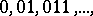over the binary alphabet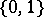. Analogously one defines rational numbers as words of a certain type over the alphabet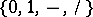. One defines the relations of order and equality on rational numbers, as well as arithmetical operations over them. A constructive sequence of natural numbers is a normal algorithm mapping every natural number into a natural number. The notion of a constructive sequence of rational numbers is treated the same way. Schemes of normal algorithms can be uniquely coded by words over the alphabet. The code of a given algorithm is called the description of the algorithm. A constructive sequence of natural numbersis called a fundamentality regulator (or modulus of convergence) of a constructive sequence of rational numbersif for any natural numberssuch that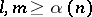one has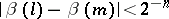. A constructive sequence of rational numbers is called fundamental if one can construct a fundamentality regulator for it.
Constructive real numbers are either rational numbers or words over the alphabet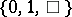of the form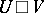(here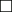plays the role of separating sign), whereis the description of a constructive sequence of rational numbers andis the description of a constructive sequence of natural numbers that is a fundamentality regulator for it. This concept of a constructive real number (introduced by N.A. Shanin, cf. , who called such numbers "FR-numberFR-numbers" or "duplex (constructive real number)duplices" ) is well compatible with the intuitive conception of computable real numbers as objects having an effective approximation by rational numbers that can be made as accurate as one pleases. For constructive real numbers one can define in a natural way the relations of order and equality, as well as the arithmetical operations (these are given by algorithms). The system of constructive real numbers with these order and equality relations and arithmetical operations turns out to be a field. Further, one may consider constructive sequences of constructive real numbers and subsequently define the same ideas as above, the concept of a fundamental constructive sequence of constructive real numbers and the concept of constructive convergence of a constructive sequence of constructive real numbers to a constructive real number. Relative to this concept of convergence, the system of constructive real numbers is complete: There is an algorithm that, starting from the description of any constructive sequence of constructive real numbersand the description of a fundamentality regulator for it, gives a constructive real number to whichconverges (constructively) (the completeness theorem for the system of constructive real numbers). By methods analogous to Cantor's method, one can also prove the constructive uncountability of the set of all constructive real numbers: There is an algorithm mapping the description of any sequence of constructive real numbers into a real number that is different (in the sense of equality of constructive real numbers) from all terms of this constructive sequence. The completeness theorem gives a remarkable similarity between the constructive and classical theories of limits. This similarity manifests itself especially clearly in questions on the convergence of some concrete sequences and series used in analysis. Along with this there are also considerable differences, manifesting themselves, e.g., in the following result of E. Specker (cf. ): It is possible to construct an increasing constructive sequence of constructive real numberssuch that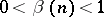always, but such thatis not fundamental (hence, does not converge (constructively) to any constructive real number). Moreover,does not contain any constructively convergent subsequence and the set of values ofdoes not attain its supremum in the constructive continuum. Another way of introducing the concept of a constructive real number is the constructivization of the notion of expansion in some base. More precisely, a constructive-ary fractionis a (normal) algorithmsuch thatis an integer and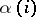for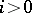is a natural number, where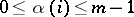(with the-ary fractionone associates the constructive sequence of constructive real numbers). Despite the simplicity of this concept of a constructive real number, it is not widely used since it has a number of essential drawbacks: e.g. the completeness theorem is not preserved, and there is no algorithm realizing the addition of any two-ary fractions.
The concept of a constructive function is a precise formulation of the intuitive notion of a pointwise-computable function over computable real numbers. A constructive function (of a real variable) is a (normal) algorithmsuch that, for any two equal constructive real numbersand, ifis applicable tothen it is applicable toandare equal constructive real numbers. The elementary functions (the exponential function, the trigonometric functions, etc.) can be introduced in terms of constructive functions, with preservation of the usual properties. Theories of differentiation, Riemann integration, etc., close to the traditional theories, can be developed for constructive real functions. Besides these, other functions not usual from the traditional point of view are possible: e.g. there has been constructed a constructive function that is everywhere defined and continuous on the unit interval but not bounded on it (cf. ). There is also no analogue in traditional analysis of the theorem stating that every constructive function is constructively continuous at every point at which it is defined (cf. ).
The system of concepts and methods of constructive analysis, having allowed one to make significant advances from the point of view of the aim 1), also turned out to be convenient for clarifying the computational relationships in analysis, since many theorems in constructive analysis are either statements on the realization of algorithms constructing objects from some initial data, or are assertions on the non-existence of such algorithms. By now (1987) the unsolvability of quite a lot of natural decidability problems in analysis has been established. Results of this type (which are completely absent in courses on traditional analysis) have a clear practical and theoretical value, since they extract potential computational features and help in giving a clear understanding of the limits of what is computable. Thus, the impossibility of the following algorithms (in the sense of some precise formulation of this concept) has been proved: 1) recognizing whether or not an arbitrary constructive real number equals zero; 2) finding for each convergent constructive sequence of rational numbers the constructive real number to which it converges; 3) finding a solution for any compatible system of linear equations (over the field of constructive real numbers); 4) finding a root of an arbitrary continuous piecewise-linear definite function; 5) finding the Riemann integral of an arbitrary continuous piecewise-linear function on the unit interval. The following theorem, which completely solves the problem on the possibility of an effective transition from one number system to another, also belongs to this circle of results. An algorithm that finds for each-ary constructive fraction the-ary constructive fraction equal to it exists if and only if the set of all prime divisors ofis contained in the set of all prime divisors of(cf. ). (In particular, there is an algorithm for going from the decimal system to the binary system, but there is no algorithm for going from the binary to the decimal system.) Theorems on the impossibility of algorithms often give rise to theorems on the existence of algorithms solving the problem considered with more complete initial data (cf. the completeness theorem for constructive real numbers and example 2)) or to an arbitrarily prescribed accuracy (e.g., it is possible to construct an algorithm that finds for each everywhere-defined definite constructive functionand eacha constructive real number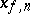such that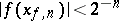). Combining such results allows one to obtain in many situations a clear view on how to pose various algorithmic problems correctly.